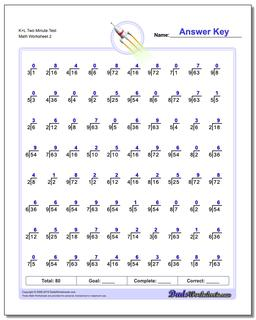One Dad. Four daughters. 9,341 worksheets... and counting!# K+L Two Minute Test (Second Worksheet)

PropertyValue
DescriptionK+L Two Minute Test: 80 problem division worksheets similar to RocketMath tests designed for two minute drills. Each of these division worksheets combines two levels from one minute tests, introducing four to five new division problems on each worksheet. (Second Worksheet)
Resource TypeWorksheet